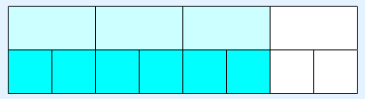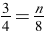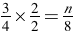# Proportions

A ratio is a comparison of two quantities that have the same unit of measure.  A proportion is an equation which states that two ratios are equal.

A proportion is a special type of equation stating that two ratios are equal.

For example,are proportions. This is the same as equivalent fractions.

A proportion is a statement that tells that two ratios are equal. An example of a proportion is. You would read this proportion like this: “3 is to 4 as 6 is to 8”. If you reduce 6/8 to lowest terms, it equals 3/4 . In the diagram below, the top row shades 3 out of 4 rectangles and the bottom row has 6 out of 8 rectangles shaded. Can you see that 3/4 in the top row is the same 6/8 in the bottom row? We can say that the proportionis a true statement.### Parts of a Proportion

There are three parts to every proportion: 2 fractions and an equal sign. Each of the fractions by itself is a ratio. The numerator of the first ratio and the denominator of the second ratio are called the extremes. The denominator of the first ratio and the numerator of the second ratio are called the means. In our example,, 3 and 8 are the extremes and 4 and 6 are the means. The cross products of all proportions are always equal. We say that “the product of the means equals the product of the extremes”. If you multiply the extremes, the product is 24. If you multiply the means, the product is also 24.

Suppose you want to know ifis a true statement. If you cross multiply, the product of the means would be 30 and the product of the extremes would be 24. Because the products are not equal, the statement is not true.

A proportion is a special type of algebraic equation that has a missing extreme or mean, such as. We would read this proportion “3 is to 4 as n is to 8″.

### How to Find the Missing Term

There are two ways to find the missing term of a proportion.

### 1. Find the equal ratio:

Sometimes it is easier for us to just find the equal ratio. For example, in the proportion, we can see that 4 was multiplied by 2 to get 8. So, we must do the same to 3.The fraction 2/2 is equal to the number 1. Multiplying by the number 1 in this form does not change the value of the fraction, just the way it looks. We find that n = 6.

On the next day we’ll learn the other way.